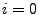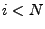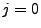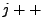Next: Output Up: Writing a New Model Previous: model_output(int flush, char *ext_)

## Macros for Referencing Field Variables in Different Numbers of Dimensions

As we mentioned above, LATTICEEASY is designed so that you can simply set the parameters of your run, including the number of dimensions, without having to worry about the detailed way these options are implemented. One way in which this is done is through the use of certain macros for referring to fields and/or indices in different number of dimensions. Typically the only place you would need to use any of these macros in creating a new model file would be when you create your own names for the fields, using the predefined macro FIELD. For example, twofldlambda.h contains the line
#define PHI FIELD(0)
which tells the program to take ``PHI" to refer to the'th field value at a particular point.

In some circumstances, however, you might want to do something more complicated with your model file. For example, if you are creating output functions specific to your model you may need to use loops and reference field values at particular points. For that reason we list here all of the macros that are predefined for this purpose. Their use should be clear from the examples you can find in the program, and in particular in twofldlambda.h.

LOOP
This is a loop over the entire array using the indices i, j, and k. For example in two dimensions this macro expands to ``for(;;) for(;;)". Note that if you use LOOP in a function you must also declare the ints i, j, and k.
FIELD(fld)
Gives the value of a field variable at a particular point referred to by the indices i, j, and k. The parameter fld specifies which field is being used. This macro is particularly useful in conjunction with the LOOP macro.
FIELDD(fld)
This is identical to FIELD except it gives the field derivative.
INDEXLIST
This is a list of arguments that can be used for a function. It expands to ``int i, ..." for one dimension, ``int i, int j, ..." for two and ``int i, int j, int k" for three. Thus a function with INDEXLIST in its argument list can take as arguments the correct number of indices for that number of dimensions, or it can take up to three indices regardless of the number of dimensions. For example the function dvdf() uses this argument and then uses the FIELD macro to refer to the field values at that point. This scheme implicitly ignores any passed indices beyond those needed for the number of dimensions being used.
FIELDPOINT(fld,i,j,k)
This macro is defined in case you need to refer to the value of a field at a specific point rather than just using the generic indices i, j, and k. In one dimension i and j are ignored and in two dimensions i is ignored.
DECLARE_INDICES
This is a list of index declarations to be put at the beginning of functions that use the LOOP macro. It declares the integers i, j, and/or k needed for the correct number of dimensions. Note that if you use any of these variable names in other ways in the function you should not use this macro but just declare them yourself.Next: Output Up: Writing a New Model Previous: model_output(int flush, char *ext_)